MapReduce is a programming paradigm that allows processing a large amount of data by initially splitting the data into blocks, sending the blocks to different clusters to perform operations, and aggregating the results.

Let’s consider a simple WordCount exercise here. From an input text, we will count how many times each word appears, and rank the final list by occurrence. MapReduce is of course not needed for such task, and a simple Python script on your computer would be fine. But what if your input text is a whole book? Thousands of books? Millions of books? …

What we’ll describe here scales easily to a large amount of data.

# The algorithm

## Step 1: Elect the Master

In the first step, we consider a set of machines which together form a cluster. We need to define which machine/node will become a Master, and which ones will become Workers.The role of the Master is to :

• split the input data on different machines
• remember which machine handles which part of the data
• handle duplicates to avoid loss of information if a worker fails
• aggregate the outputs and sort the final list

## Step 2: Split the input data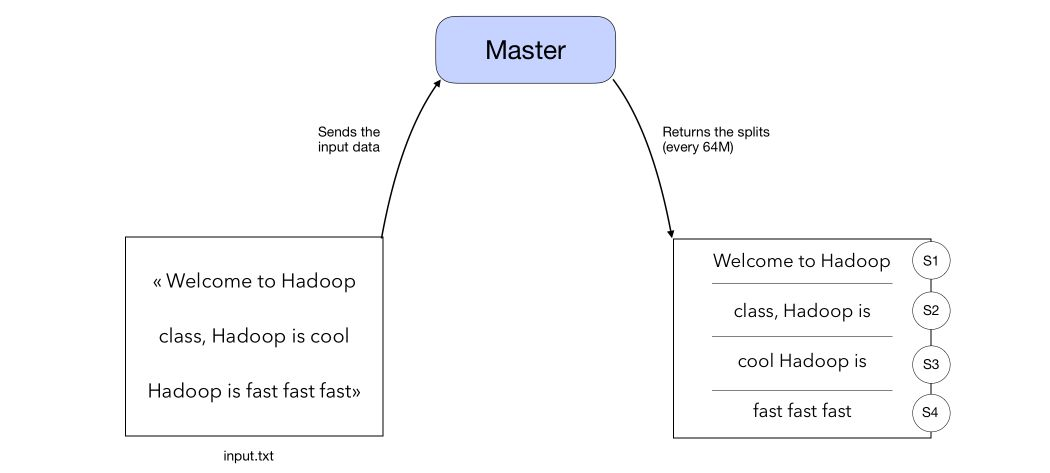The input data is split into blocks of 64Mb. A 1Ko text file might use a whole 64Mb data node inside a cluster, so it is preferable to have rather big files. Optimization tools are provided by Hadoop.

## Step 3: Map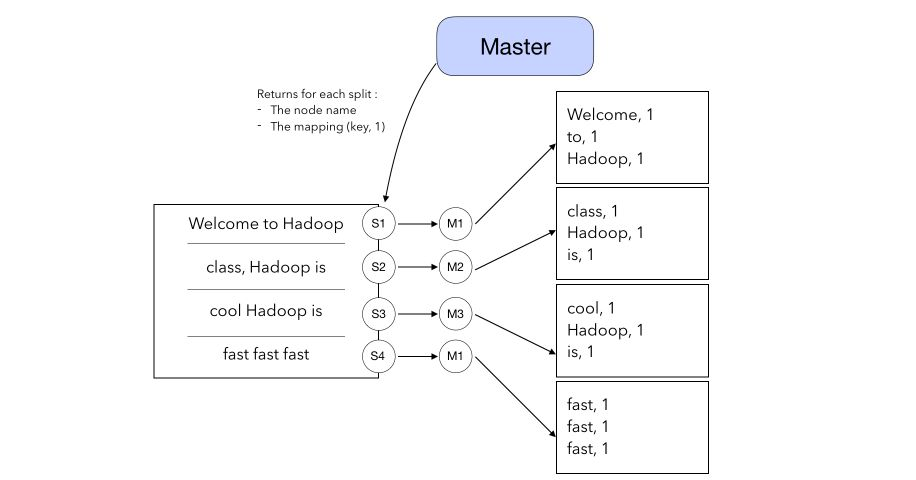In the Map, the Master returns for each split :

• the node name to which the split should be sent
• the mapping (Key, value) where value is equal to 1 for every word of the split

## Step 4: Shuffle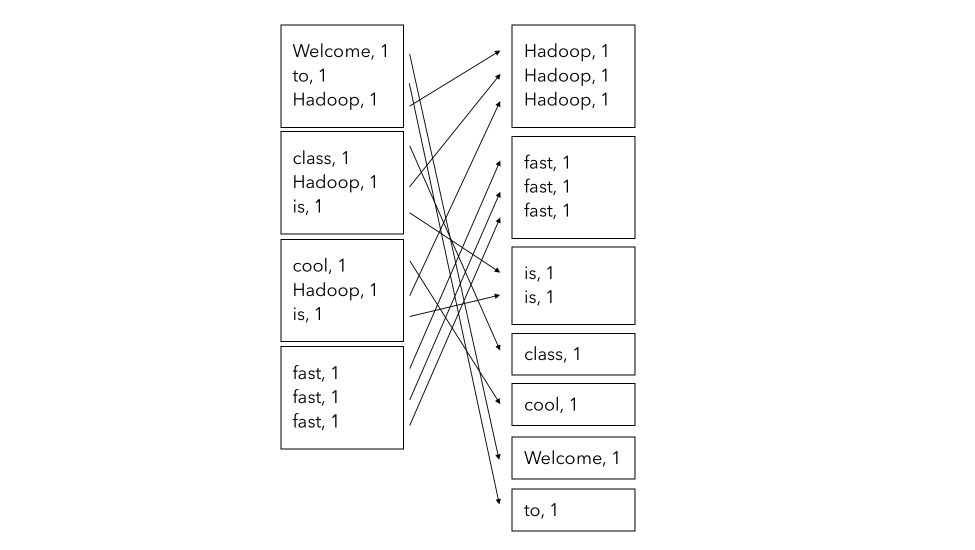In the Shuffle step, the Map-Reduce algorithm groups the words by similarity (group a dictionary by key). It is called Shuffle because the initial splits are no longer used.

## Step 5: Reduce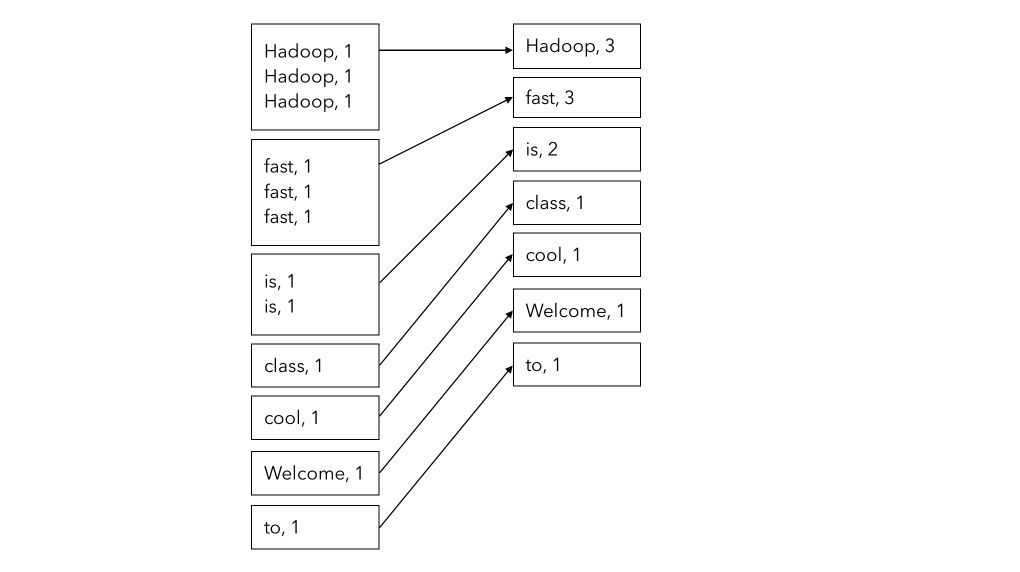In the Reduce step, we simply compute the sum of all values for a given key. This is simply the sum of all the 1’s of the key. Remember that this step is still parallelized, so the Master still handles how the different key-value attributes are stored and computed across the different machines.

## Step 6: Sorting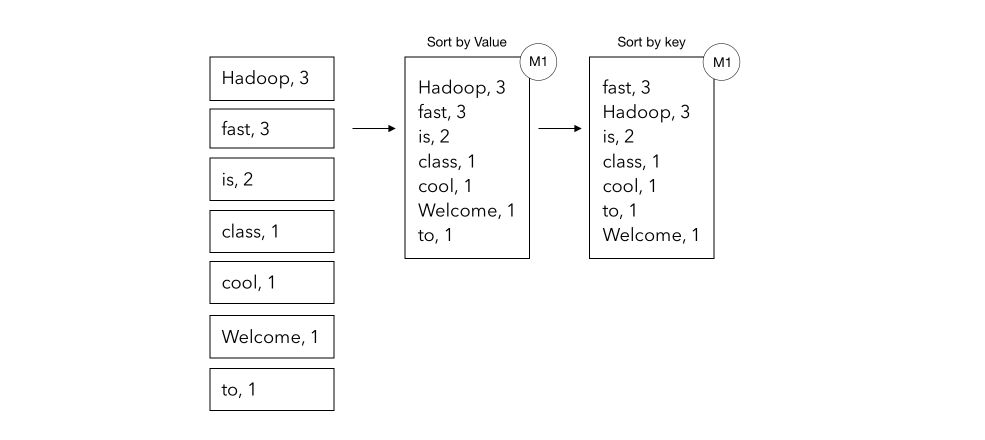The last step is to sort by (first) value, then by key, and return the final list as a `.txt` file.

## The Big Picture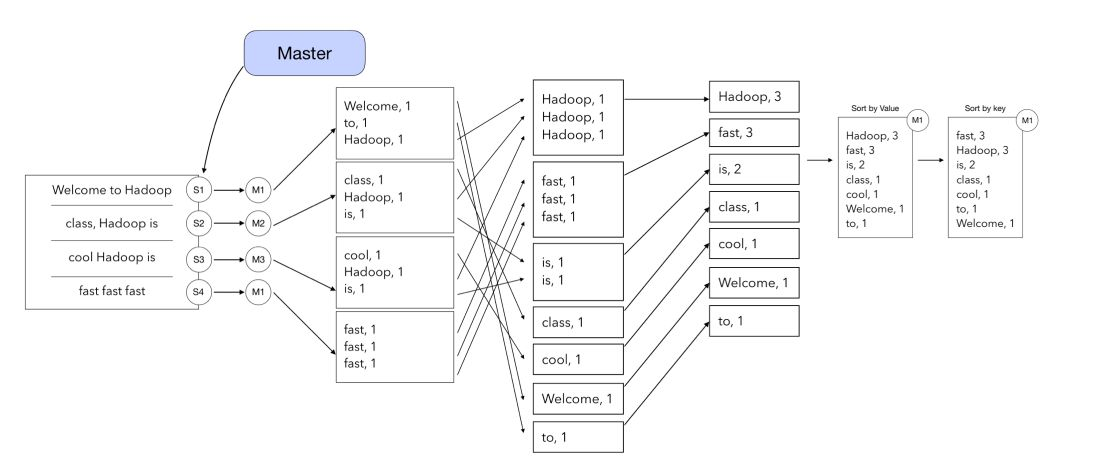# Replication factor and cluster types

## Replication factor

As we discussed above, Hadoop MapReduce can handle the failure of a node. When does failure occur?

• Connection with a node is lost (network)
• The node itself breaks (over 5’000 machines working full time in a data center, expect several to break down each day)

How do we handle failure?

• In the Map step, the splits are not sent to simply one machine, but each machine stores several data splits, in case other machines would break.
• This is called the replication factor.
• In case the Master fails, we have a second Master up and running!

By default, every data block is replicated 3 times, so distributed 3 times on different DataNodes, and each NameNode is replicated 2 to 3 times.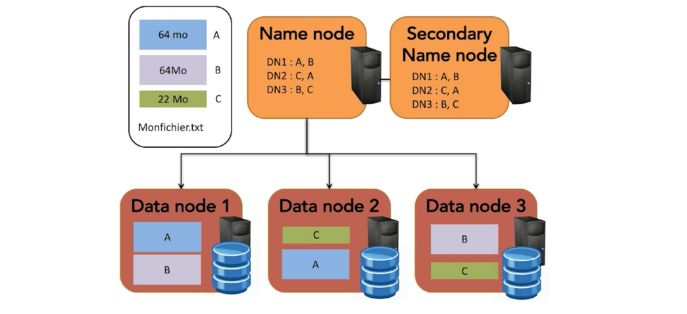## Cluster types

As a recap, there are 3 types of nodes on a Hadoop Cluster :

• Edge node: This is an entry point for the client, as we do not want him to connect straight to the Master.
• Master node: Hosts all servers and administration of the MapReduce algorithm.
• Worker nodes: Store the data and the different computations. If we face a higher demand, we simply dynamically add workers.

### Other operations

We presented a toy example using WordCount. We could also try to build Map, Combine and Reduce functions for other tasks :

#### Find Minimum (or maximum) :

• Map : Identity function `f(x) = (x, 1)`
• Combine: Take the smallest key among each chunk (We could drop duplicates using the value associated with each key)
• Reduce: Return the smallest key

#### Find the mean :

• Map : Identity function `f(x) = (x, 1)`
• Combine : Take the sum of key and sum of values : `f( (a,b), (c,d) ) = (a+c, b+d)`
• Reduce : Return the sum of keys / sum of values

#### Find the median :

We make the assumption that there is a limited number of different numbers :

• Map : Identity function within a list `f(x) = [(x, 1)]`
• Combine : Take the sum of key and sum of values : `f( [(x, 1)], [(x, 1)], [(y, 1)] ) = [(x, 2), (y, 1)]`
• Reduce : Sort and return the median

Conclusion: I hope this high-level overview was clear and helpful. I’d be happy to answer any question you might have in the comments section.

Categories:

Updated: